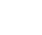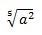Home Latest News Office Insiders on MAC in the Insider Slow ring now have support...

# Office Insiders on MAC in the Insider Slow ring now have support for LaTeX equation and more

0Office Insiders on MAC in the Insider Slow ring can take advantage of the LaTex equation support. Users can notate vectors, nary, fractions, radicals, etc.

Depending on your preferred input format, you can create equations in Word in either one of UnicodeMath or LaTeX formats by selecting the format from the Equations tab.### Create fractions in linear formats

• Enter your equation using Alt + = on the keyboard.
• Choose Convert and select professional to build your typed fractions to their Professional form into subscripts, or use Ctrl + =. You can similarly convert an equation back down to a linear format with Ctrl + Shift + =.Examples### LaTex equation editing examples

LaTeX equation editing supports most of the common LaTeX mathematical keywords. To create a 3×3 matrix equation in the LaTeX format, type the following into a math zone:

A=\{\matrix{a&b&c\\d&e&f\\g&h&j}\}

This will build into the following professional equation:Here are some other examples of LaTeX expressions that can be built-up into a professional format.Most LaTeX expressions are supported in this new feature to Word; a list of exceptions is provided below for LaTeX keywords that are not currently supported.Some LaTeX expressions take a slightly different syntax than might be expected.

For example, LaTeX matrices are often created using the following syntax:

\begin{matrix} a & b \\ c & d \end{matrix}

However \begin{} and \end{} keywords are not supported in Word, so instead, a LaTeX matrix input takes simply \matrix{} and would look like:

\matrix{a & b \\ c & d}

### UnicodeMath editing examples

UnicodeMath resembles real mathematical notation the most in comparison to all of the math linear formats, and it is the most concise linear format, though some may prefer editing in the LaTeX input over UnicodeMath since that is widely used in academia.

You can type most equations in UnicodeMath quickly by using Math AutoCorrect codes. For example, to align an equation array, you can use @ and &, as in the following:

\eqarray(x+1&=2@1+2+3+y&=z@3/x&=6)<space>

which resolves to:Here are some other examples:

Example Vectors UnicodeMath format Built-up format (abc)\vec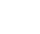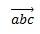(abc)\hat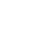\rect(a/b)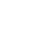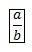(a+b/c)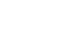{a+b/c}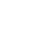{a/b\vbarx+y\vbar}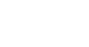a/(b+c)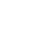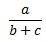_a^bx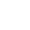lim_(n->\infty)n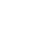(\matrix(a&b@&c&d))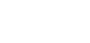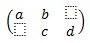\iint_(a=0)^\inftya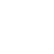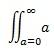\overbar(abc)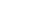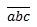\overbrace(a+b)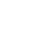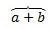\sqrt(5&a^2)